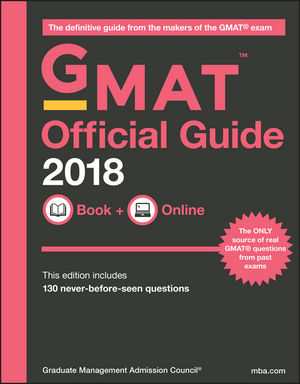GMAT Ladder Program coaches leverage the Facilitative Approach in order to assess the strengths and areas for improvement of each student. Students arrive at each new session with a list of the homework questions that they either answered incorrectly, or that took them ‘too long’ to answer. While students work through questions on the board, their MathSP coach facilitates a discussion that enables students to find the best solutions and understand their mistakes. Through the expert guidance of the MathSP coach and the input of their peers, students work through missed questions to not only correct their mistakes but to also gain a solid understanding of the concepts at the center of each GMAT question.

### The Ladder Program Course Information

• Intense foundation review of important Math concepts (listed below)
• Real GMAT problems tackled using our proven method, the SP Method
• Organization of problems by concept to promote Concept-based Learning
• Extensive homework assignment assigned each session
• Homework review at the start of each session utilizing the Facilitative Approach
• Online practice tests throughout the course to encourage familiarity with the CAT format
• In-class timed practice stints to understand the importance of timing on the GMAT

### The Ladder Program Course Outline

The standard Ladder Program course consists of eight 3-hr sessions. Each of the Quantitative problems in “The Official Guide for GMAT Review” is assigned for homework and discussed in class throughout the eight sessions.  This text is written by the Graduate Management Admission Council, GMAC, the organization that writes and administers the official GMAT.  Thus, mastering the problems in this text in an appropriate time frame is essential as the problems in this text are real GMAT problems similar to those students see on their official GMAT.  MathSP ensures that you understand the Math concepts tested on the GMAT and how to apply those concepts and strategies to real GMAT problems.

Sessions are organized as follows:

• Session 1:  Arithmetic Part I
• Session 2:  Arithmetic Part II
• Session 3:  Algebra Part I
• Session 4:  Algebra Part II and Statistics
• Session 5:  Geometry Part I
• Session 6:  Geometry Part II
• Session 7:  Special Strategies and Time-saving Tips
• Session 8:  Integrated Reasoning Strategies and Problem-solving

The Quantitative section of the GMAT tests your knowledge of a finite number of Math concepts. Mastering these concepts is critical to scoring your highest on the GMAT. In the Ladder Program, our coaches teach the Math concepts listed below that are required for your success on the GMAT.

The concepts can be categorized into three groups:

• arithmetic
• algebra & statistics
• geometry

Concepts include:

##### Arithmetic

Number Theory, Properties of Integers, Fractions, Decimals, Prime Numbers, Even and Odd Numbers, Positive and Negative Numbers, Consecutive Integers, Number Lines, Digits, Factors and Multiples, Factor Trees, Order of Operations, Percentages, Ratios, Proportions, Squares and other Powers, Square Roots, Data Analysis, Interpretation of Graphs and Tables, Operations on Rational Numbers, Work Rates

##### Algebra and Statistics

Exponents and Powers, Solving Equations, Substitution, FOIL, Factoring, Absolute Value, Distance Rates, Inequalities, Applied Algebra, Translating Math Sentences into Equations, Mean, Median, Linear Functions, Quadratic Functions, Symbols as Representations of Operations, Probability, Permutations, Combinations, Sets, Standard Deviation

##### Geometry

Lines and Angles (Vertical, Supplementary, Complementary, Alternate Interior, Parallel, Perpendicular, Tangent), Circles (Radius, Diameter, Area, Circumference, Arc Length, Inscribed), Triangles (Special Right Triangles, Pythagorean Theorem, Inequality Theorem, Area, Perimeter, Equilateral, Isosceles), Quadrilaterals (Area, Perimeter), Integrated Shapes, Shaded Regions, Volume (Cube, Rectangular Prism, Cylinder), Coordinate Geometry

### The Ladder Program Course Materials

##### Course Materials Include:• “The Official Guide for GMAT Review,” by the GMAC
• “The Official Guide for GMAT Quantitative Review,” by the GMAC (optional)
• Math foundation review books (optional)